# #459 OpAmp/TwinTeeOscillator

A twin-tee sine wave oscillator using an TL072 Op-Amp, running in the audio spectrum.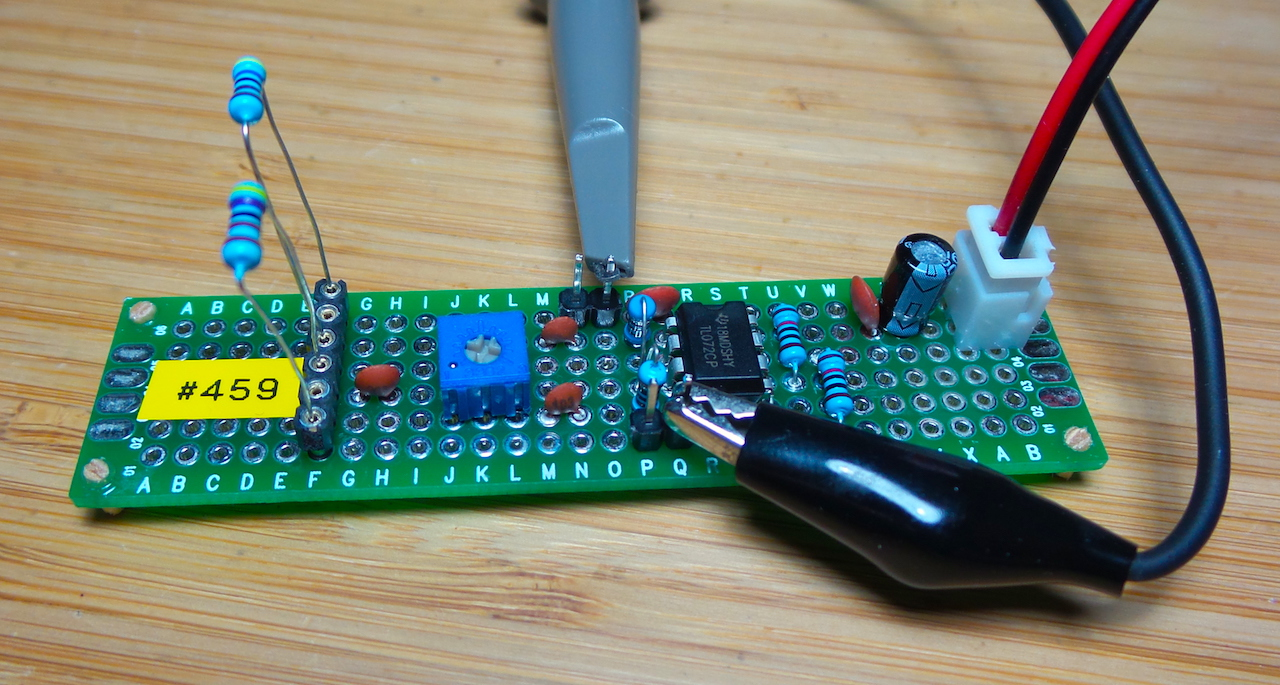A twin-tee filter in the feedback loop of an op-amp can be used to generate a sine wave.

• one tee comprises an R-C-R low-pass filter
• the other is a C-R-C high-pass filter.

Together, these circuits form a notch filter which is tuned at the desired frequency of oscillation.

## Design Guidelines

For oscillations to be sustained, x>=2, where:

``````x = C2/C1 = R1/R2
``````

And the frequency is then given as:

``````frequency = 1/(2πRC)
``````

I’m using a TL072:

• one op-amp unit is used to establish a buffered AC ground at VCC/2
• the other is for the oscillator

R2 is a pot so that it can be adjusted to be ~ R1/2 and thus allow the circuit to oscillate depending on the R1 values used.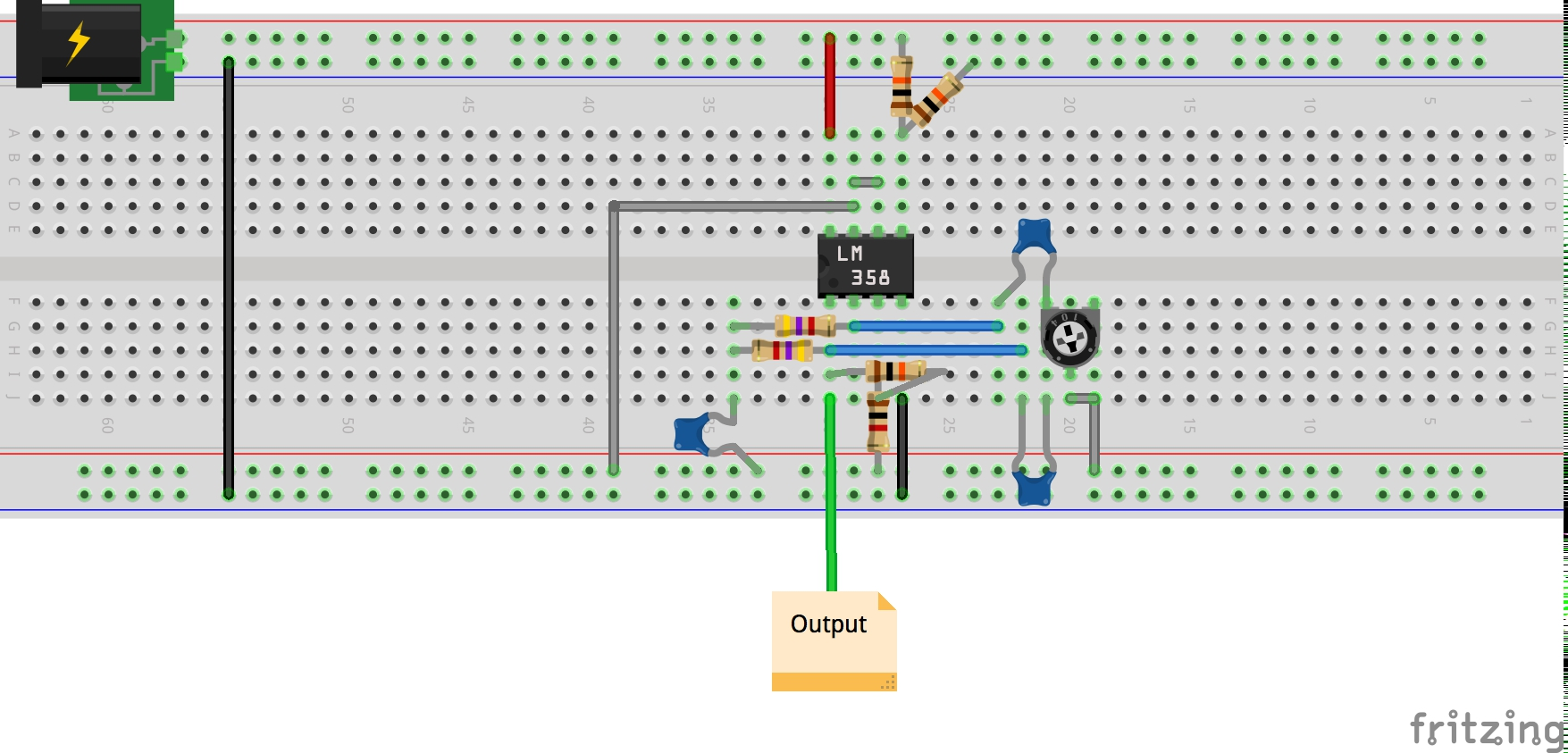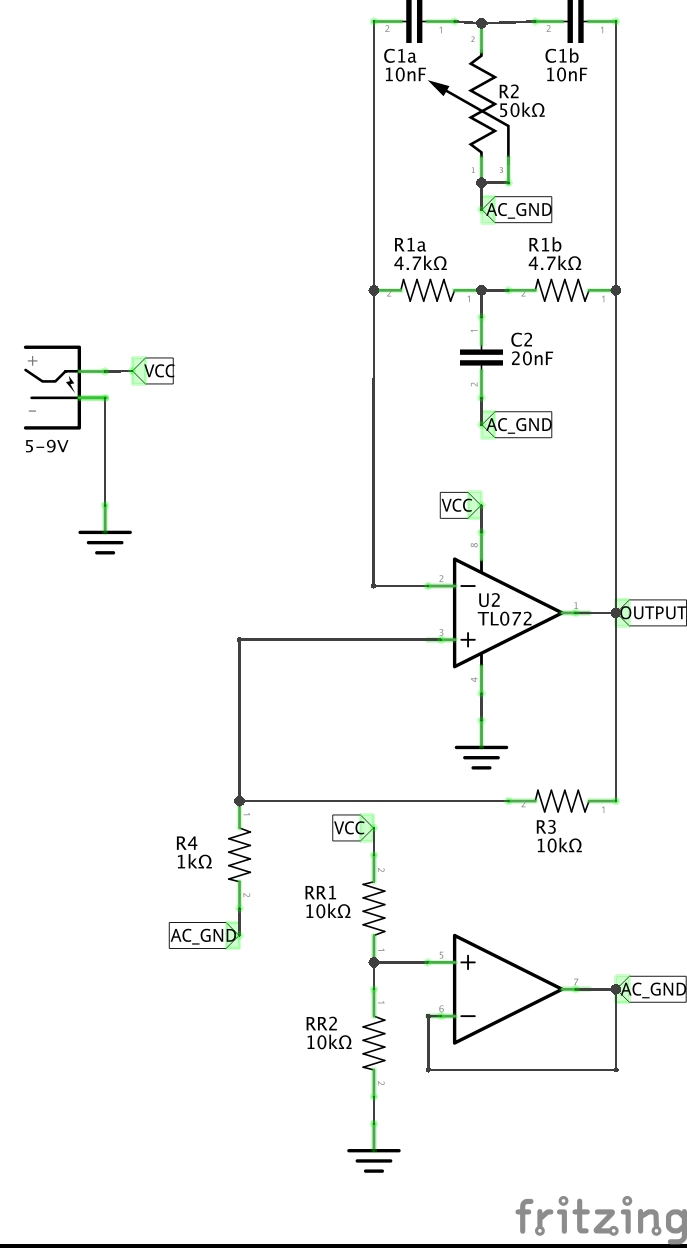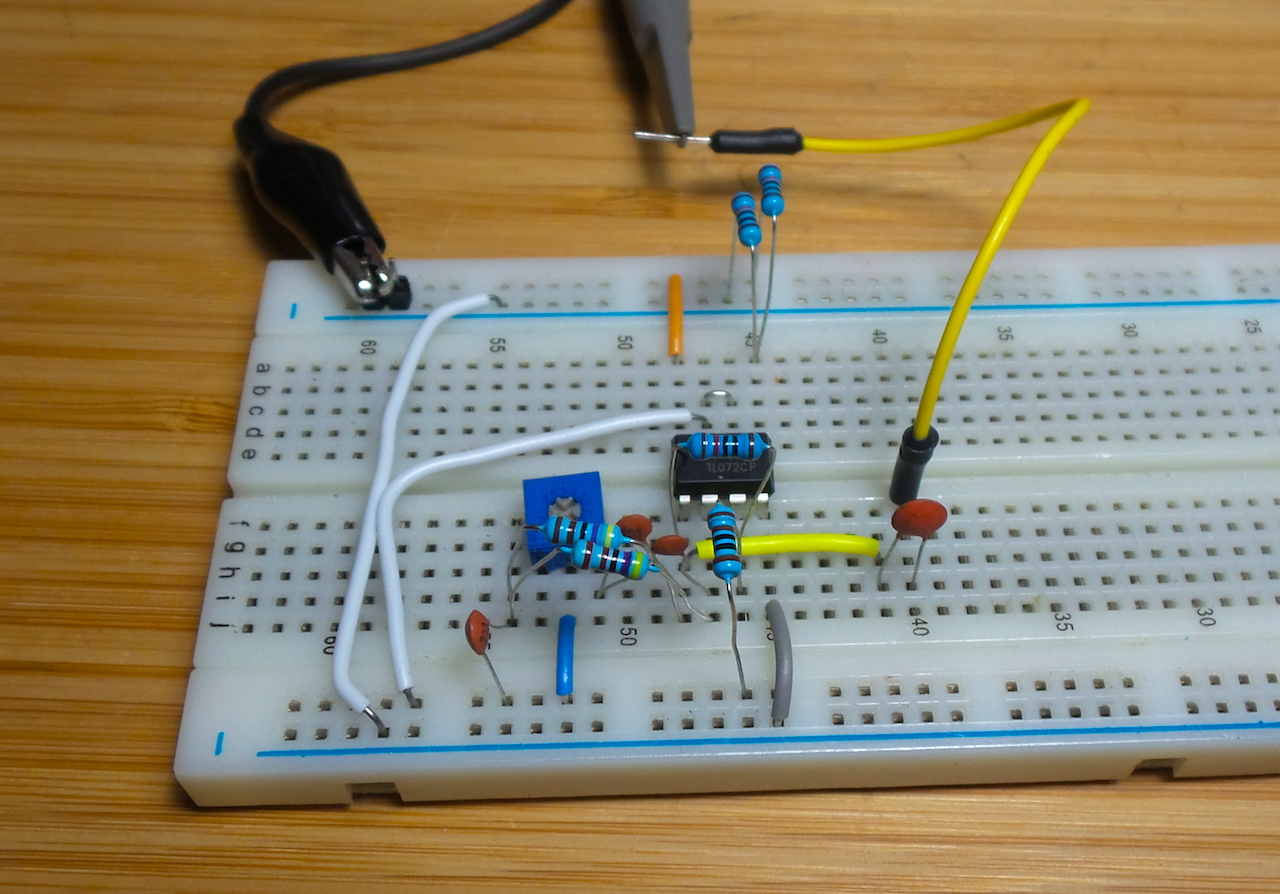With a 9V supply and R1 = 4.7kΩ, I get oscillation at about 3.238kHz: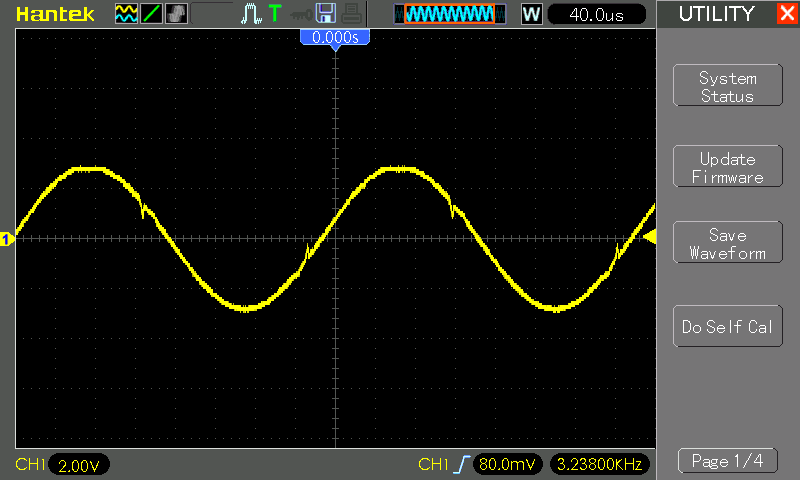## Protoboard Construction

For some more reliable measurements with various resistor values, I put the circuit on some protoboard. Here’s the basic layout I used. It includes:

• connections for substituting R1 values
• pins for output and capacitively coupled output (via 100nF capacitor)
• pins for ground and AC ground
• some capacitors for power supply smoothing (100nF and 100µF across the power supply)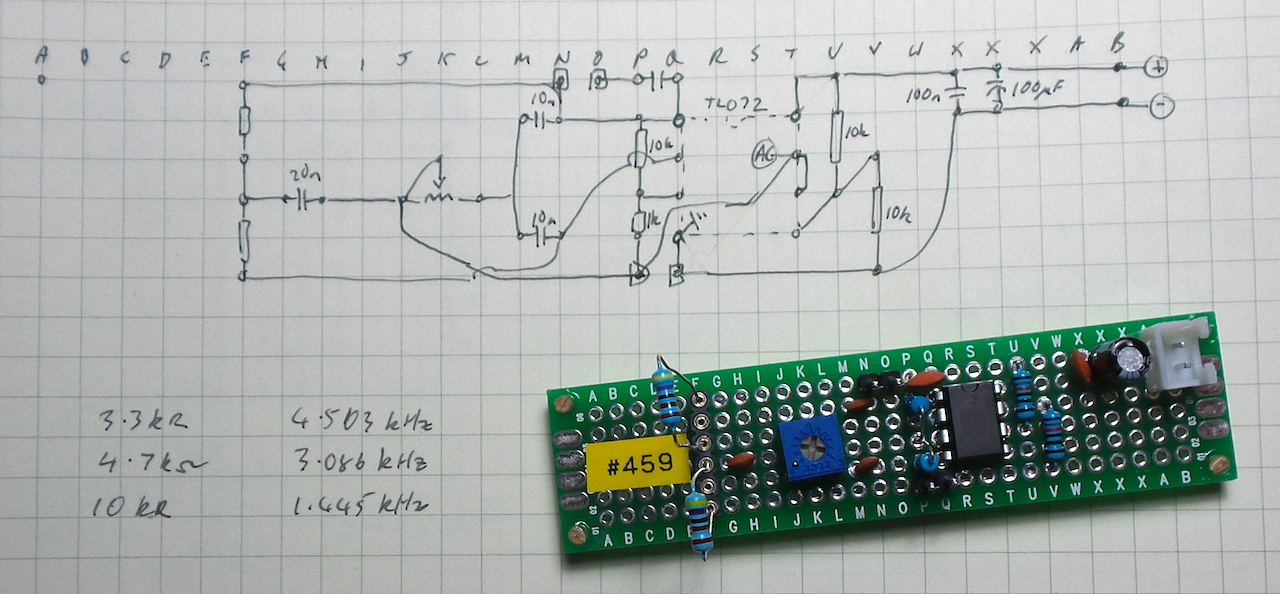With a 5V supply and R1 = 4.7kΩ, I get oscillation at about 3.084kHz. The wave is not a perfect sine wave, with two main distortions present:

• noticable cross-over distortion in both directions
• clipping at the top of the wave if R2 not adjusted correctly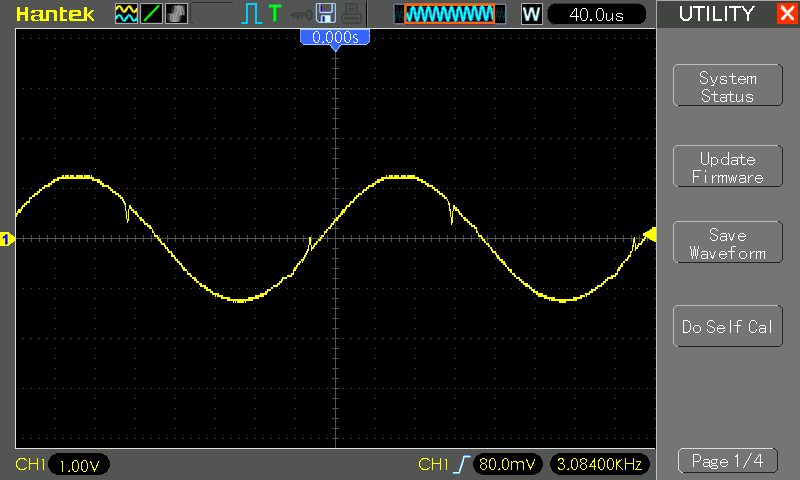Some measurements with a selection of R1 values:

R1 Expected Frequency Actual Frequency
3.3kΩ 4.823kHz 4.503kHz
4.7kΩ 3.386kHz 3.084kHz
10kΩ 1.592kHz 1.445kHz